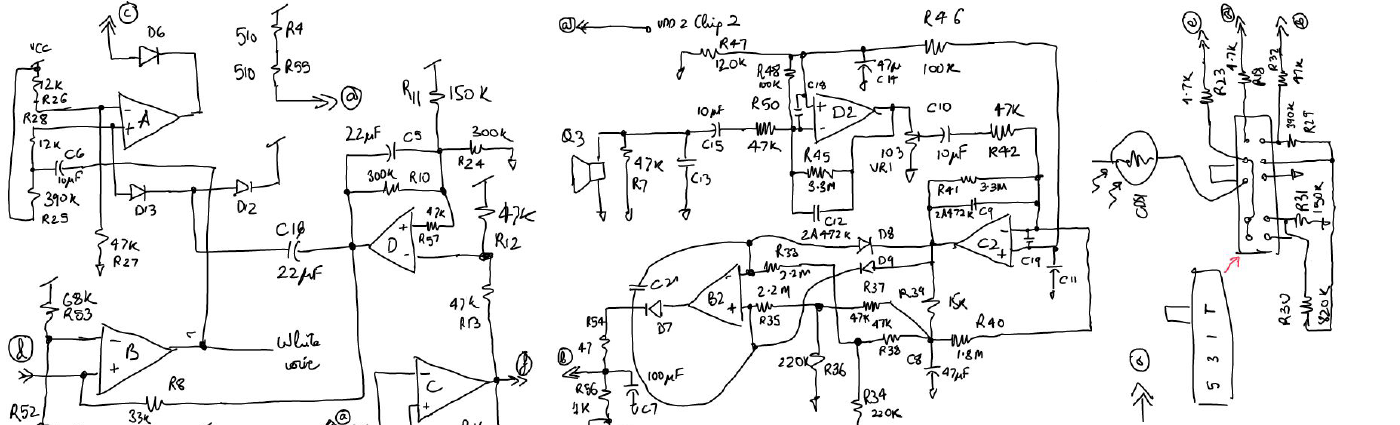# Ballpark NumbersThere are a lot of process numbers which are quite common across processes and good to have handy to know what can be achieved in a design.

• The variation of an untrimmed bandgap made using Vbe and resistors is usually +/-3% and we add a trim range of +/- 4.5 to 5%
• For an untrimmed bandgap the temperature variation of the voltage at extreme corners may be like +/- 0.5% (~6mV) or +/-1% (12mV if taken to 150C) from the room voltage, while that of a trimmed bandgap may be <+/-0.2% (2mV).
• Capacitances vary about +/- 16%
• MOS gate cpacitance is more tightly controlled and is about +/-10%
• Resistor variation depends on the width of the resistor and polycrystalline resistors can vary anywhere from +/- 50% to +/-10% (PI)
• Bipolar Vbe varies about +/- 2.5% over process corners (room temperature)

• High voltage FETs (100s of volts) usually have very high drain capacitances. This causes the dain node to rise slowly but when the FET needs to turn ON it wastes a lot of energy stored in the drain cap.

# Transformers

• For small power supply adapters the transformers made to switch at 100KHz and close frequencies usually have capacitance like in the 10s of pico farads so have parasitic loading of 100pF on the switching transistor can be reasonable.

# Noise and Dynamic Range

• Noise spectral density at room temperature for a 1K resistor is 4nV/√Hz
• Noise spectral density at room temperature for a 50 ohm resistor is 0.9nV/√Hz
• For 1pF capacitor √(kT/C) at room temperature is 64uV-rms
• For 1000pF capacitor √(kT/C) at room temperature is 2uV-rms
• For supplies of <3V and Cmax<100pF the maximum limit of the dynamic range at room is 104dB. Derived as:
$$V_{max}(rms)=V_{DD}/2 1/√2$$
$$V_n(rms)=√{α{k_BT}/C}$$

$$\text"Dynamic Range"={V_{max}(rms)}/{V_n(rms)}={V_{DD}√C}/√{8αk_BT}$$

$$DR=20log_{10}(V_{DD}√{C/α})+75dB\text" withC in pF"$$

# Opamp design

As a rule of thumb the highest unity gain frequency you can try to achieve for a opamp is ft/10. ft is the unity gain transition frequency of the device in the process at which the current gain from input to output is 1.

# Filters

• Inductors on CMOS can be in the range of <15nH with Q of <7.
• For Active filter design on chip any poles with a Q > 4 are not a good idea since it will make the transfer function vary too much due to component variation. (Q of poles is determined by the RC time constant of the Active filter integrator)